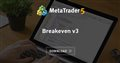# Breakeven mystery

I found nice little EA only for modifying SL and TP here https://www.mql5.com/en/code/23037

EA moves positions to Breakeven+profit.

Now when I test it I see that if there is 1 BUY position open, TP is correctly where it should be(let say 30 pips).
But as soon as there is coming more BUY positions in, TP is not coming closer to trades but goes further and further.
I need to get that 30 pips profit from all BUY positions together.
I also noticed that if I use EA without trading function it works correctly with manually opened trades.
So, what I miss here?

```#property link      "blog forex"
#property version   "1.000"
//---
#include <Expert\Money\MoneyFixedMargin.mqh>
CPositionInfo  m_position;                   // trade position object
CSymbolInfo    m_symbol;                     // symbol info object
CAccountInfo   m_account;                    // account info wrapper
CDealInfo      m_deal;                       // deals object
COrderInfo     m_order;                      // pending orders object
CMoneyFixedMargin *m_money;
//--- input parameters
input int Delta=30;
//---
ulong          m_slippage=10;                // slippage
bool     UseSound     = true;
bool     gbNoInit     = false;           // Флаг неудачной инициализации
string   SoundSuccess = "ok.wav";        // Звук успеха
string   SoundError   = "timeout.wav";   // Звук ошибки
int      NumberOfTry  = 3;
input ulong    m_magic=15489;                // magic number
datetime m_prev_bars                = 0;
//+------------------------------------------------------------------+
//| Expert initialization function                                   |
//+------------------------------------------------------------------+
int OnInit()
{
//---
if(!m_symbol.Name(Symbol())) // sets symbol name
return(INIT_FAILED);
RefreshRates();
//---
return(INIT_SUCCEEDED);
}
//+------------------------------------------------------------------+
//| Expert deinitialization function                                 |
//+------------------------------------------------------------------+
void OnDeinit(const int reason)
{
//---
}
//+------------------------------------------------------------------+
//| Expert tick function                                             |
//+------------------------------------------------------------------+
void OnTick()
{
//---
datetime time_0=iTime(Symbol(),PERIOD_CURRENT,0);
if(time_0==m_prev_bars)
return;
m_prev_bars=time_0;
//---
MqlRates rates[];
ArraySetAsSeries(rates,true);
int copied=CopyRates(_Symbol,_Period,0,5,rates);
//---
if(rates.close > rates.open && rates.close > rates.open && rates.close > rates.open && rates.close > rates.open)
{
}

double M=0.0,MM=0.0,Prof=0.0,LL=0.0;
int total=0;
for(int i=PositionsTotal()-1; i>=0; i--) // returns the number of current positions
if(m_position.SelectByIndex(i))     // selects the position by index for further access to its properties
if(m_position.Symbol()==m_symbol.Name())
{
Prof+=m_position.Commission()+m_position.Swap()+m_position.Profit();
total++;
LL=LL+m_position.Volume();
if(m_position.PositionType()==POSITION_TYPE_SELL)
LL=LL-m_position.Volume();
}
if(total==0)
{
Comment("");
return;
}
M=BEZ(); // Уровень безубытка для BUY
if(!RefreshRates())
return;
Comment("Уровень безубытка ",M,"+",Delta,"\n",
"Надо пройти  ",DoubleToString(MathAbs(M-m_symbol.Bid())/m_symbol.Point(),0)," points","\n",
" Лот=",DoubleToString(LL,2)," лот","\n",
"Общий профит  ",DoubleToString(Prof,2));

if(LL<0) // Если больше SELL То безубыток - Delta ниже Bid и наоборот
{
MM=M-Delta*m_symbol.Point();  // Если больше SELL
for(int i=PositionsTotal()-1; i>=0; i--) // returns the number of current positions
if(m_position.SelectByIndex(i)) // selects the position by index for further access to its properties
if(m_position.Symbol()==m_symbol.Name())
{
Modify(-1,MM,-1);
if(m_position.PositionType()==POSITION_TYPE_SELL && !CompareDoubles(m_position.TakeProfit(),MM,m_symbol.Digits()))
Modify(-1,-1,MM);
}
}
if(LL>0) // Если больше BUY То безубыток + Delta выше Bid
{
for(int i=PositionsTotal()-1; i>=0; i--) // returns the number of current positions
if(m_position.SelectByIndex(i)) // selects the position by index for further access to its properties
if(m_position.Symbol()==m_symbol.Name())
{
Modify(-1,-1,MM);
if(m_position.PositionType()==POSITION_TYPE_SELL && !CompareDoubles(m_position.TakeProfit(),MM,m_symbol.Digits()))
Modify(-1,MM,-1);
}
}
}
//+------------------------------------------------------------------+
//| Функция подсчёта безубытка                                       |
//+------------------------------------------------------------------+
double BEZ()
{
double B2_B=0.0,B2_S=0.0,B2_LB=0.0,B2_LS=0.0,BSw=0.0,SSw=0.0;

for(int i=PositionsTotal()-1; i>=0; i--) // returns the number of current positions
if(m_position.SelectByIndex(i)) // selects the position by index for further access to its properties
if(m_position.Symbol()==m_symbol.Name())
{
{
BSw=+m_position.Commission()+m_position.Swap(); // Total profit
}
if(m_position.PositionType()==POSITION_TYPE_SELL)
{
B2_S=((B2_S*B2_LS)+(m_position.PriceOpen()*m_position.Volume()))/(B2_LS+m_position.Volume());
B2_LS=B2_LS+m_position.Volume();
SSw+=m_position.Commission()+m_position.Swap();
}
}
double M2B=0.0,M2S=0.0,M=0.0;
if(B2_LB>B2_LS) // Идём вверх
{
for(int J2=0; J2<10000; J2++)
{
M2B=J2*B2_LB*10;
M2S=((B2_B-B2_S+0*m_symbol.Point())/m_symbol.Point()+J2)*(B2_LS*(-10));
if(M2B+M2S+BSw+SSw>=0)
{
M=NormalizeDouble(B2_B+J2*m_symbol.Point(),m_symbol.Digits());
break;
}
}
}
if(B2_LS>B2_LB) //  Идём вниз
{
for(int J3=0; J3<10000; J3++)
{
M2S=J3*B2_LS*10;
M2B=((B2_B-B2_S+0*m_symbol.Point())/m_symbol.Point()+J3)*(B2_LB*(-10));
if(M2S+M2B+BSw+SSw>=0)
{
M=NormalizeDouble(B2_S-J3*Point(),m_symbol.Digits());
break;
}
}
}
//---
return(M);
}
//+------------------------------------------------------------------+
//| Refreshes the symbol quotes data                                 |
//+------------------------------------------------------------------+
bool RefreshRates(void)
{
//--- refresh rates
if(!m_symbol.RefreshRates())
{
Print("RefreshRates error");
return(false);
}
//--- protection against the return value of "zero"
return(false);
//---
return(true);
}
//+------------------------------------------------------------------+
//| Compare doubles                                                  |
//+------------------------------------------------------------------+
bool CompareDoubles(double number1,double number2,int digits)
{
digits--;
if(digits<0)
digits=0;
if(NormalizeDouble(number1-number2,digits)==0)
return(true);
else
return(false);
}
//+------------------------------------------------------------------+
//|                                                                  |
//+------------------------------------------------------------------+
void Modify(double pp=-1,double sl=0,double tp=0)
{
double op=0.0,pa=0.0,pb=0.0,os=0.0,ot=0.0;

if(pp<=0)
pp=m_position.PriceOpen();
if(sl<0)
sl=m_position.StopLoss();
if(tp<0)
tp=m_position.TakeProfit();

op=m_position.PriceOpen();
os=m_position.StopLoss();
ot=m_position.TakeProfit();

if(!CompareDoubles(pp,op,m_symbol.Digits()) ||
!CompareDoubles(sl,os,m_symbol.Digits()) ||
!CompareDoubles(tp,ot,m_symbol.Digits()))
{
m_symbol.NormalizePrice(sl),
m_symbol.NormalizePrice(tp)))
{
if(UseSound)
PlaySound(SoundError);
Sleep(1000*10);
}
else
{
if(UseSound)
PlaySound(SoundSuccess);
}
}
}
//+------------------------------------------------------------------+
//| Calculate all positions                                          |
//+------------------------------------------------------------------+
int CalculateAllPositions()
{
int total=0;

for(int i=PositionsTotal()-1; i>=0; i--)
if(m_position.SelectByIndex(i)) // selects the position by index for further access to its properties
if(m_position.Symbol()==m_symbol.Name() && m_position.Magic()==m_magic)
total++;
//---
return(total);
}
//+------------------------------------------------------------------+
```Breakeven v3
• www.mql5.com
Moving positions to breakeven

Learn to code or hire a freelancer
Reason: